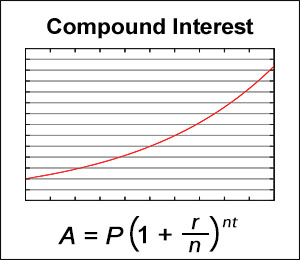# Compound Interest Calculator

Compound interest is the interest that is calculated from the initial principal and the accumulated interest. Due to compound interest, the principal is able to grow at an increasing rate like shown in the graph shown below.

If the "Compounded Times" is below 12, the output will show data each time interest was compounded; however, if the value is above 12, it will calculate accordingly but will only show a maximum of 12 times per year in the output.

The "Balance" is rounded up to the cent whereas the interest is rounded to the fourth decimal place.

See APY Interest Calculator to take into account monthly deposits.

Compound Interest Formula

A = P*(1+(r/n))^(n*t)

Where "A" is the balance after n years, "P" is the principal amount, "r" is the annual interest rate, "t" is the number of years, and "n" is the number of times interest is compounded in a year.CC BY-SA 3.0 - Original Graph by Autopilot.

### Input

 Principal Amount(P): \$ Annual Interest(r): % Years(t): years Compounded Times(n) times/year